### 介绍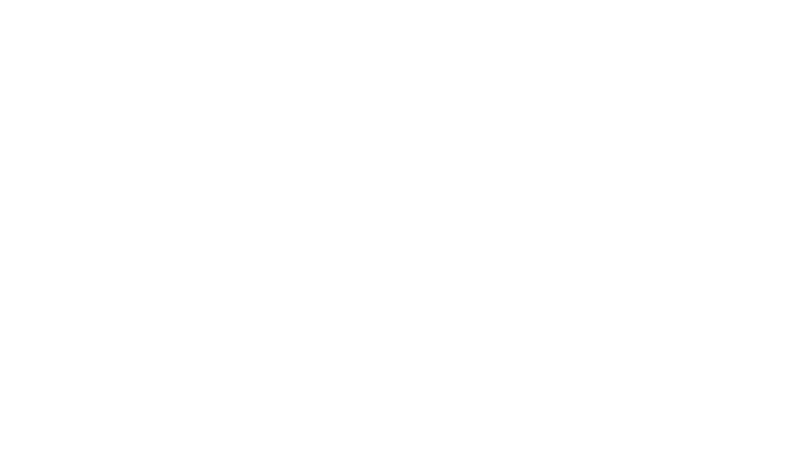### 目录

1. 什幺是RetinaNet

1. RetinaNet的需求

RetinaNet的架构

1. 骨干网

1. 对象分类子网

1. 对象回归子网

Focal Loss

1. 收集数据

1. 创建数据集

1. 模特训练

1. 模型测试

### 什幺是RetinaNet

RetinaNet是一种最好的单目标检测模型，已被证明能很好地处理密集和小尺度的物体。由于这个原因，它已经成为一个流行的目标检测模型。

### RetinaNet的架构

1. 主干网络（自底向上的路径和具有横向连接的自上而下的路径）

1. 目标分类子网

1. 目标回归子网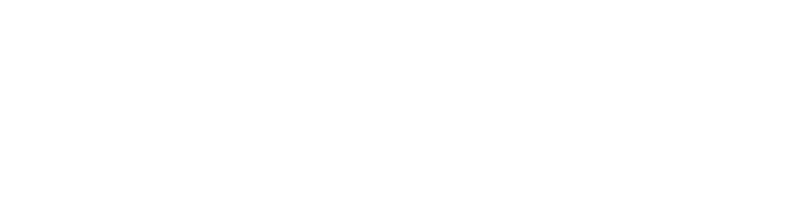#### 1.主干网络

1. 自底向上路径:自底向上路径(例如，ResNet)用于特征提取。因此，它计算不同比例的特征图，而不考虑输入图像的大小。

1. 具有横向连接的自上而下的路径：在自上而下的路径上，从更高的金字塔级别对空间上较粗糙的特征图进行上采样，横向连接将具有相同空间大小的自顶向下和自底向上的层合并在一起。较高层次的特征图往往具有较小的分辨率，但语义上更强。因此，更适合于检测较大的物体；相反，来自较低级特征图的网格单元具有高分辨率，因此更擅长检测较小的对象。因此，结合自上而下的路径及其与自底向上的路径的横向连接，不需要太多额外的计算，因此生成的特征图的每个级别在语义和空间上都可以很强。 因此，该体系结构是规模不变的，并且可以在速度和准确性方面提供更好的性能。

### Focal Loss

Focal Loss（FL）是Cross-Entropy Loss（CE）的改进版本，它通过为困难的或容易错误分类的示例（即具有嘈杂纹理或部分对象或我们感兴趣的对象的背景）分配更多权重来尝试处理类不平衡问题 ，并简化简单的示例（即背景对象）。

1- pt代表交叉熵损失，可调聚焦参数≥0。 RetinaNet物体检测方法使用焦距损失的α平衡变体，其中α= 0.25，γ= 2效果最佳。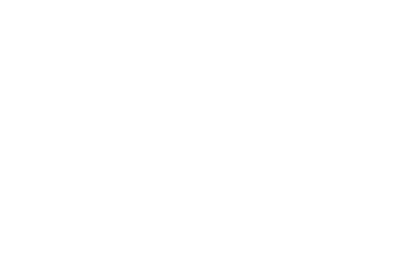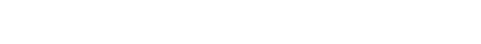1. 当示例分类错误且pt小时，调制因子接近1并且不影响损失。

1. 当pt→1时，因子变为0，并且可以很好地权衡分类良好的示例的损失。

1. Focal loss γ平滑地调整了简单示例的权重。 随着γ的增加，调制因子的作用也同样增加。 （经过大量实验和试验，研究人员发现γ= 2最有效）

### 利用RetinaNet模型建立口罩检测器

#### 创建数据集

`pip install labelImg`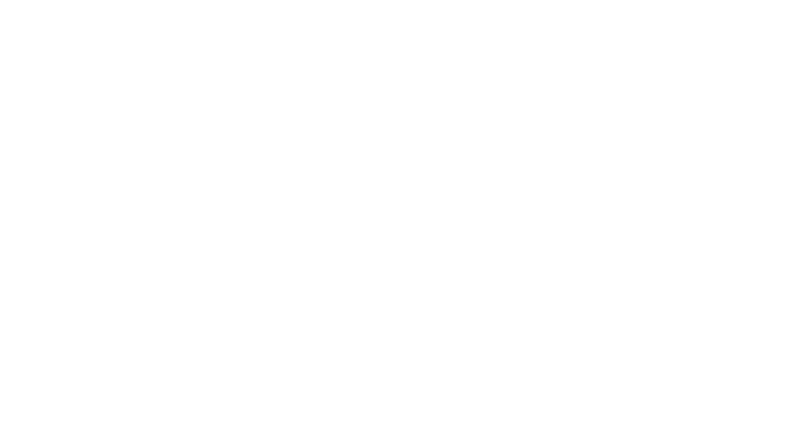#### 第1步：克隆并安装keras-retinanet

```import os
print(os.getcwd())```

```git clone https://github.com/fizyr/keras-retinanet.git
%cd keras-retinanet/
!pip install .
!python setup.py build_ext --inplace```

#### 第2步：导入所有必要库

```import numpy as np
import shutil
import pandas as pd
import os, sys, random
import xml.etree.ElementTree as ET
import pandas as pd
from os import listdir
from os.path import isfile, join
import matplotlib.pyplot as plt
from PIL import Image
import requests
import urllib
from keras_retinanet.utils.visualization import draw_box, draw_caption , label_color
from keras_retinanet.utils.image import preprocess_image, resize_image```

#### 第3步：导入JPEG和xml数据

```pngPath='C:/Users/PraveenKumar/RetinaNet//maskDetectorJPEGImages/'
data=pd.DataFrame(columns=['fileName','xmin','ymin','xmax','ymax','class'])
os.getcwd()
##读所有文件
allfiles = [f for f in listdir(annotPath) if isfile(join(annotPath, f))]```

```#读取所有pdf文件的图像，然后在文本存储在临时文件夹
for file in allfiles:
#print(file)
if (file.split(".")=='xml'):
tree = ET.parse(annotPath+file)
root = tree.getroot()
for obj in root.iter('object'):
cls_name = obj.find('name').text
xml_box = obj.find('bndbox')
xmin = xml_box.find('xmin').text
ymin = xml_box.find('ymin').text
xmax = xml_box.find('xmax').text
ymax = xml_box.find('ymax').text
#通过添加字典在空数据框架中追加行
data = data.append({'fileName': fileName, 'xmin': xmin, 'ymin':ymin,'xmax':xmax,'ymax':ymax,'class':cls_name}, ignore_index=True)
data.shape```

#### 第4步：编写一个函数来显示训练数据集上的边界框

```# 随机选取图像
filepath = df.sample()['fileName'].values
# 获取此图像的所有行
df2 = df[df['fileName'] == filepath]
im = np.array(Image.open(filepath))
# 如果有PNG的话，它会有alpha通道
im = im[:,:,:3]
for idx, row in df2.iterrows():
box = [
row['xmin'],
row['ymin'],
row['xmax'],
row['ymax'],
]
print(box)
draw_box(im, box, color=(255, 0, 0))
plt.axis('off')
plt.imshow(im)
plt.show()
show_image_with_boxes(data)```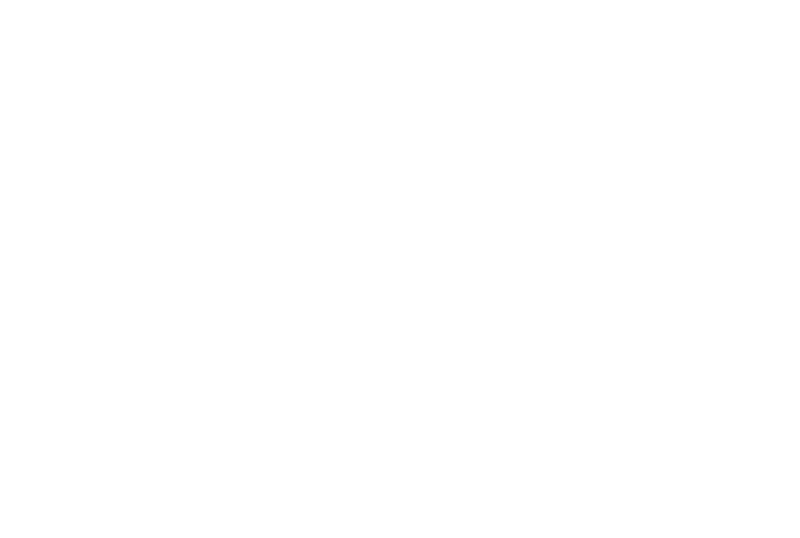```#检查少量数据记录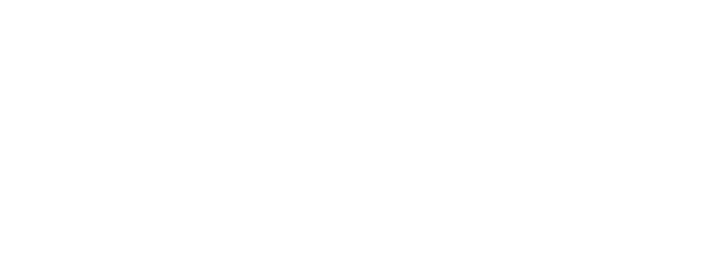```#定义标签并将其写入文件
for i, class_name in enumerate(classes):
f.write(f'{class_name},{i}\n')
if not os.path.exists('snapshots'):
os.mkdir('snapshots')```

```URL_MODEL = 'https://github.com/fizyr/keras-retinanet/releases/download/0.5.1/resnet50_coco_best_v2.1.0.h5'
urllib.request.urlretrieve(URL_MODEL, PRETRAINED_MODEL)```

#### 第5步：训练RetinaNet模型

```#把你的训练数据路径和文件放在训练数据的标签上
!keras_retinanet/bin/train.py --freeze-backbone \ --random-transform \ --weights {PRETRAINED_MODEL} \ --batch-size 8 \ --steps 500 \ --epochs 15 \ csv maskDetectorData.csv maskDetectorClasses.csv```

```python keras_retinanet/bin/train.py --freeze-backbone
--random-transform \
--weights {PRETRAINED_MODEL}
--batch-size 8
--steps 500
--epochs 15

1. freeze-backbone:冻结主干层，当我们使用小数据集时特别有用，以避免过拟合

1. random-transform:随机变换数据集以获得数据增强

1. weights:使用一个预先训练好的模型(您自己的模型或者Fizyr发布的模型)初始化模型

1. batch-size:训练批量大小，值越高，学习曲线越平滑

1. step:迭代的步数

1. epochs:迭代的次数

1. csv:上面的脚本生成的注释文件

#### 第6步：载入训练模型

```from glob import glob
model_paths = glob('snapshots/resnet50_csv_0*.h5')
latest_path = sorted(model_paths)[-1]
print("path:", latest_path)

from keras_retinanet import models
model = models.convert_model(model)
label_map = {}
row = line.rstrip().split(',')
label_map[int(row)] = row```

#### 第7步：利用训练模型进行预测

```#写一个函数，从你的数据集中随机选择一个图像，并预测使用训练模型。
def show_image_with_predictions(df, threshold=0.6):
# 随机选择一个图像
row = df.sample()
filepath = row['fileName'].values
print("filepath:", filepath)
# 获取此图像的所有行
df2 = df[df['fileName'] == filepath]
im = np.array(Image.open(filepath))
print("im.shape:", im.shape)
# 如果有一个PNG，它会有alpha通道
im = im[:,:,:3]
# 画出真实盒子
for idx, row in df2.iterrows():
box = [
row['xmin'],
row['ymin'],
row['xmax'],
row['ymax'],
]
print(box)
draw_box(im, box, color=(255, 0, 0))
### 画出预测 ###
# 获取预测
imp = preprocess_image(im)
imp, scale = resize_image(im)
boxes, scores, labels = model.predict_on_batch(
np.expand_dims(imp, axis=0)
)
# 标准化框坐标
boxes /= scale
# 循环得到每个预测
for box, score, label in zip(boxes, scores, labels):
# 分数是排序的，所以一旦我们看到分数低于阈值，我们就可以退出
if score < threshold:
break
box = box.astype(np.int32)
color = label_color(label)
draw_box(im, box, color=color)
class_name = label_map
caption = f"{class_name} {score:.3f}"
draw_caption(im, box, caption)
score, label=score, label
plt.axis('off')
plt.imshow(im)
plt.show()
return score, label
plt.rcParams['figure.figsize'] = [20, 10]```

```#可以根据你的业务需求随意更改阈值
label=show_image_with_predictions(data, threshold=0.6)``````#可以根据你的业务需求随意更改阈值
label=show_image_with_predictions(data, threshold=0.6)```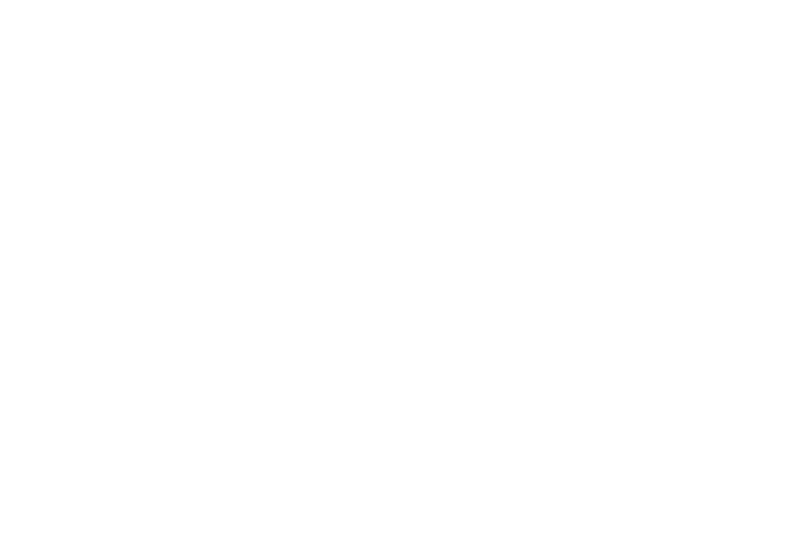```#可以根据你的业务需求随意更改阈值
label=show_image_with_predictions(data, threshold=0.6)```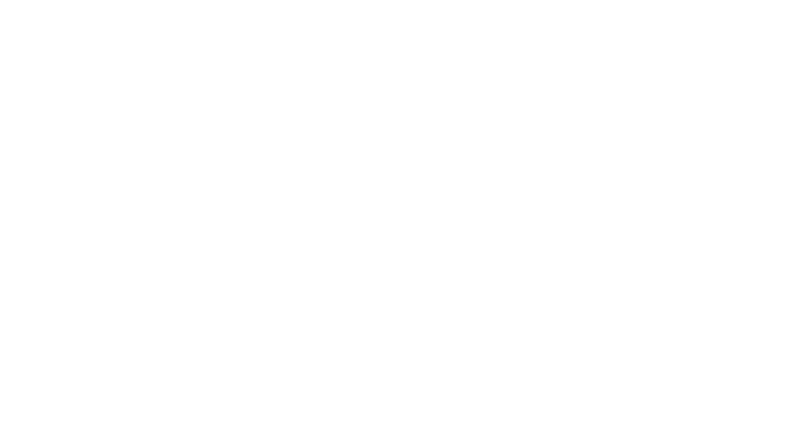```#可以根据你的业务需求随意更改阈值
label=show_image_with_predictions(data, threshold=0.6)```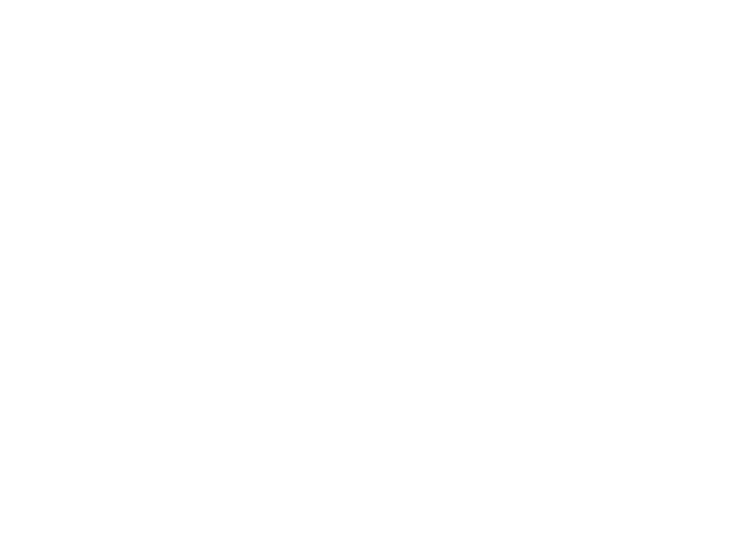```#可以根据你的业务需求随意更改阈值
label=show_image_with_predictions(data, threshold=0.6)```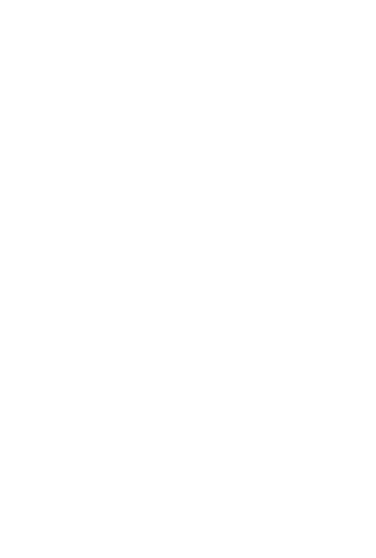```#可以根据你的业务需求随意更改阈值
score, label=show_image_with_predictions(data, threshold=0.6)```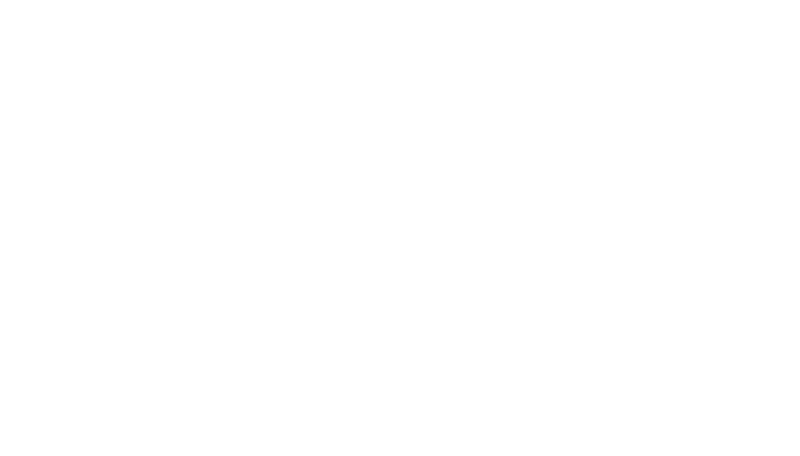```#可以根据你的业务需求随意更改阈值
score, label=show_image_with_predictions(data, threshold=0.6)```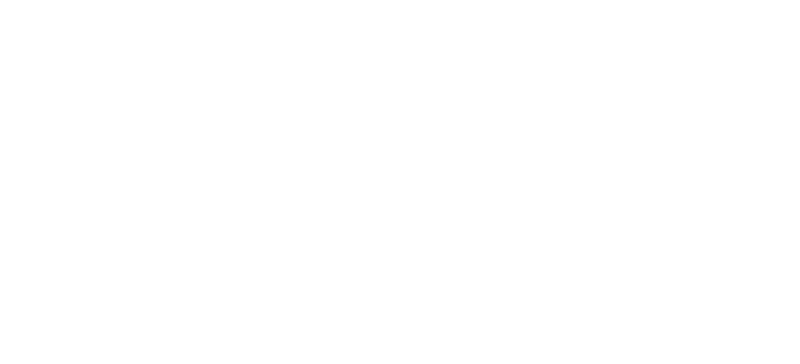```#可以根据你的业务需求随意更改阈值
score, label=show_image_with_predictions(data, threshold=0.6)```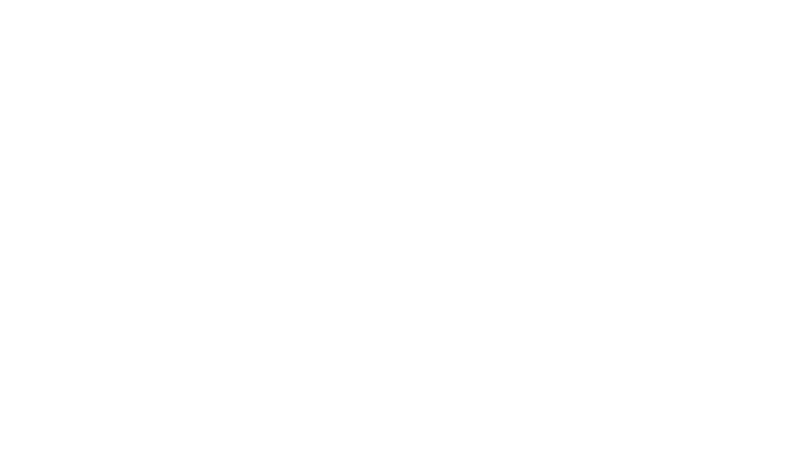### 最后说明

RetinaNet是一个功能强大的模型，使用Feature Pyramid Networks＆ResNet作为其骨干。 我能够通过非常有限的数据集和极少的迭代（每个迭代有500个步长，共6次迭代）获得口罩检测器的良好结果。当然你也可以更改阈值。

1. 确保你训练你的模型至少20次迭代，以获得好的结果。

1. 一个好的想法是提交使用RetinaNet模型构建口罩检测器的方法。 人们总是可以根据业务需求调整模型，数据和方法。